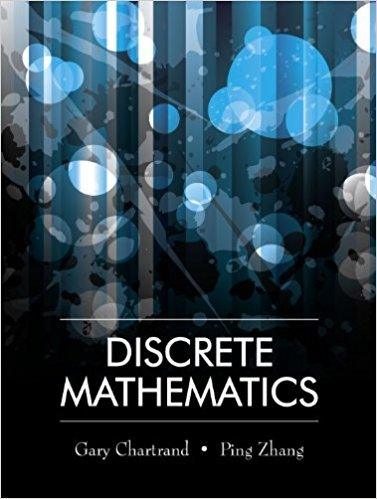×
×

# Solutions for Chapter 2: Sets## Full solutions for Discrete Mathematics | 1st Edition

ISBN: 9781577667308Solutions for Chapter 2: Sets

Solutions for Chapter 2
4 5 0 295 Reviews
30
2
##### ISBN: 9781577667308

This expansive textbook survival guide covers the following chapters and their solutions. Discrete Mathematics was written by and is associated to the ISBN: 9781577667308. This textbook survival guide was created for the textbook: Discrete Mathematics, edition: 1. Since 27 problems in chapter 2: Sets have been answered, more than 13010 students have viewed full step-by-step solutions from this chapter. Chapter 2: Sets includes 27 full step-by-step solutions.

Key Math Terms and definitions covered in this textbook

A sequence of steps (end of Chapter 9) to solve positive definite Ax = b by minimizing !x T Ax - x Tb over growing Krylov subspaces.

• Covariance matrix:E.

When random variables Xi have mean = average value = 0, their covariances "'£ ij are the averages of XiX j. With means Xi, the matrix :E = mean of (x - x) (x - x) T is positive (semi)definite; :E is diagonal if the Xi are independent.

• Echelon matrix U.

The first nonzero entry (the pivot) in each row comes in a later column than the pivot in the previous row. All zero rows come last.

• Ellipse (or ellipsoid) x T Ax = 1.

A must be positive definite; the axes of the ellipse are eigenvectors of A, with lengths 1/.JI. (For IIx II = 1 the vectors y = Ax lie on the ellipse IIA-1 yll2 = Y T(AAT)-1 Y = 1 displayed by eigshow; axis lengths ad

• Exponential eAt = I + At + (At)2 12! + ...

has derivative AeAt; eAt u(O) solves u' = Au.

• Fibonacci numbers

0,1,1,2,3,5, ... satisfy Fn = Fn-l + Fn- 2 = (A7 -A~)I()q -A2). Growth rate Al = (1 + .J5) 12 is the largest eigenvalue of the Fibonacci matrix [ } A].

• Fourier matrix F.

Entries Fjk = e21Cijk/n give orthogonal columns FT F = nI. Then y = Fe is the (inverse) Discrete Fourier Transform Y j = L cke21Cijk/n.

• Hessenberg matrix H.

Triangular matrix with one extra nonzero adjacent diagonal.

• Hypercube matrix pl.

Row n + 1 counts corners, edges, faces, ... of a cube in Rn.

• lA-II = l/lAI and IATI = IAI.

The big formula for det(A) has a sum of n! terms, the cofactor formula uses determinants of size n - 1, volume of box = I det( A) I.

• Linear combination cv + d w or L C jV j.

• Markov matrix M.

All mij > 0 and each column sum is 1. Largest eigenvalue A = 1. If mij > 0, the columns of Mk approach the steady state eigenvector M s = s > O.

• Multiplier eij.

The pivot row j is multiplied by eij and subtracted from row i to eliminate the i, j entry: eij = (entry to eliminate) / (jth pivot).

• Nullspace N (A)

= All solutions to Ax = O. Dimension n - r = (# columns) - rank.

• Right inverse A+.

If A has full row rank m, then A+ = AT(AAT)-l has AA+ = 1m.

• Row picture of Ax = b.

Each equation gives a plane in Rn; the planes intersect at x.

• Row space C (AT) = all combinations of rows of A.

Column vectors by convention.

• Schur complement S, D - C A -} B.

Appears in block elimination on [~ g ].

• Singular Value Decomposition

(SVD) A = U:E VT = (orthogonal) ( diag)( orthogonal) First r columns of U and V are orthonormal bases of C (A) and C (AT), AVi = O'iUi with singular value O'i > O. Last columns are orthonormal bases of nullspaces.

• Toeplitz matrix.

Constant down each diagonal = time-invariant (shift-invariant) filter.

×